# Calculating the Future Value of a Single Amount (FV)

If we know the single amount (PV), the interest rate (i), and the number of periods of compounding (n), we can calculate the future value (FV) of the single amount. Calculations #1 through #5 illustrate how to determine the future value (FV) through the use of future value factors.

### Calculation #1

You make a single deposit of \$100 today. It will remain invested for 4 years at 8% per year compounded annually. What will be the future value of your single deposit at the end of 4 years?

The following timeline plots the variables that are known and unknown: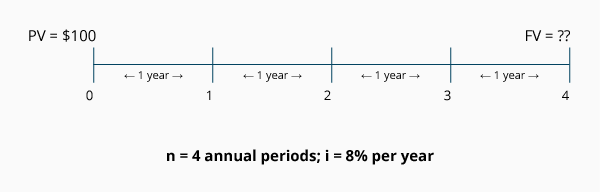Calculation using an FV factor: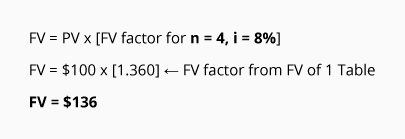At the end of 4 years, you will have \$136 in your account.

### Calculation #2

Paul makes a single deposit today of \$200. The deposit will be invested for 3 years at an interest rate of 10% per year compounded semiannually. What will be the future value of Paul's account at the end of 3 years?

The following timeline plots the variables that are known and unknown: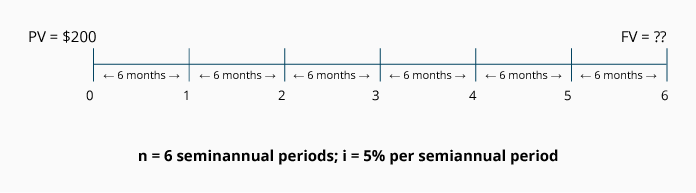Because the interest is compounded semiannually, we convert 3 years to 6 semiannual periods, and the annual interest rate of 10% to the semiannual rate of 5%.

Calculation using an FV factor: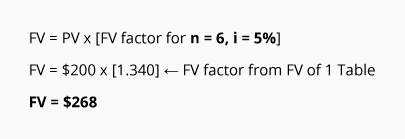At the end of 3 years, Paul will have \$268 in his account.

### Calculation #3

Sheila invests a single amount of \$300 today in an account that will pay her 8% per year compounded quarterly. Compute the future value of Sheila's account at the end of 2 years.

The following timeline plots the variables that are known and unknown: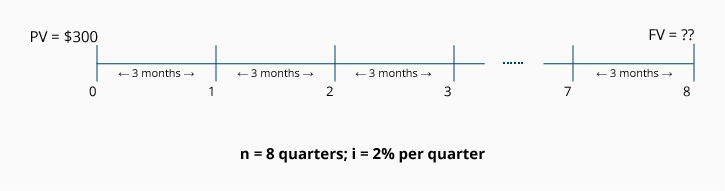Because interest is compounded quarterly, we convert 2 years to 8 quarters, and the annual rate of 8% to the quarterly rate of 2%.

Calculation using an FV factor: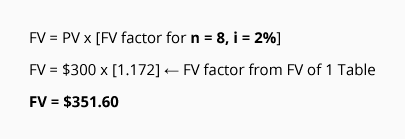At the end of 2 years, Sheila will have \$351.60 in her account.

### Calculation #4

You invest \$400 today in an account that earns interest at a rate of 12% per year compounded monthly. What will be the future value at the end of 2 years?

The following timeline plots the variables that are known and unknown: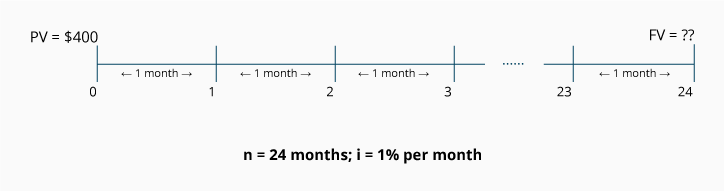Because the interest is compounded monthly, we convert 2 years to 24 months, and the annual rate of 12% to the monthly rate of 1%.

Calculation using an FV factor: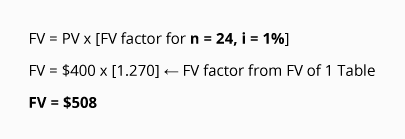At the end of 2 years, you will have \$508 in your account.